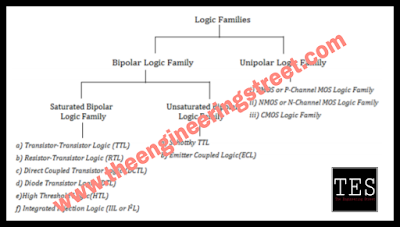# Logic Families - Bipolar Logic And Unipolar Logic Families

## Logic Families - Bipolar Logic And Unipolar Logic Families

Logic Families - Bipolar Logic And Unipolar Logic Families :

### Basically logic family divided into two parts i.e, Bipolar logic and Unipolar logic families as shown in below figure.Classification of logic families

#### A) Bipolar Logic Families:

Bipolar means two polarity.

It mainly uses bipolar devices like diodes, transistors in addition to passive elements like resistors and capacitors.

Bipolar logic families classified as Saturated bipolar logic family and Unsaturated bipolar logic family.

#### i) Saturated Bipolar Logic Family:

In this family the transistors used in ICs are driven into saturation.

Examples:

a) Transistor-Transistor Logic (TTL)
b) Resistor-Transistor Logic (RTL)
c) Direct Coupled Transistor Logic (DCTL)
d) Diode Transistor Logic (DTL)
e)High Threshold Logic(HTL)
f) Integrated Injection Logic (IIL or I2L)

Here some short information about above classification.

#### A) TTL:

Transistor Transistor Logic

It is a logic family built from bipolar junction transistor(BJT).

Its name signifies that transistors perform both the logic function ( first "transistor") and the     amplifying function ( second "transistor").

it is the same naming convention used in resistor–transistor logic (RTL) and diode transistor   logic (DTL).

#### B) RTL:

As its name suggests, RTL circuits mainly consists of resistors and transistors that comprises RTL devices.
The basic RTL device is a NOR gate, shown in figure below.

#### C) DTL:

The diode-transistor logic, also termed as DTL, replaced RTL family because of greater fan-out capability and more noise margin.

RTL allows the construction of NOR gates easily, but NAND gates are relatively more difficult to get from RTL.

DTL, however, allows the construction of simple NAND gates from a single transistor, with the help of several diodes and resistors.

#### D) Direct Coupled Transistor Logic (DCTL) :

is also called as Integrated injection logic (I2C) circuit. It uses no biasing and loading resistors .

Resistors require lot of power and space on an IC chip.

ii)Unsaturated bipolar logic family:

In this family the transistors used in ICs are not driven into saturation.

Examples:

a) Schottky TTL
b) Emitter Coupled Logic(ECL)

Schottky TTL is combination of schottky diode and transistor.

It uses schottky transistor ,response of output is batter than the TTL .Also operates at high speed than TTL

Emitter-coupled logic (ECL) is a high-speed IC bipolar transistor logic family.

ECL is sometimes called current-steering logic (CSL), current-mode logic (CL) or current-switch emitter-follower (CSEF) logic.

#### B) Unipolar Logic Families:

Unipolar means only one polarity.

It mainly uses Unipolar devices like MOSFETs in addition to passive elements like resistors and capacitors.

These logic families have the advantages of high speed and lower power consumption than Bipolar families.

These are classified as:

i) PMOS or P-Channel MOS Logic Family
ii) NMOS or N-Channel MOS Logic Family
iii) CMOS Logic Family

As shown in figure classification of logic families.

#### CMOS :

It is stands for complementary metal oxide semiconductor.

The basic building blocks in CMOS logic circuits are MOS transistors. i.e, Metal Oxide Semiconductor.

A MOS transistor contains 4 terminals named as Gate(G), Source(S), Drain(D) and Substrate(Sb).

In these 4 terminals Gate is an insulating terminal.

So no conduction will takes place between remaining two(S and D) terminals. Hence it has highest resistance between Source and Drain.

CMOS is combination of PMOS&NMOS. Basically PMOS&NMOS are connected in complementry connection to produce a CMOS.

It contains pull-up and pull-down networks.

Pull-up network contains PMOS transistor and pull-down network consists of NMOS Transistor.

In PMOS , the substrate terminal is n-type semiconductor. the channel is  p-type semiconductor.

Whereas ,in NMOS the substrate terminal is p-type and the channel is n-type.

To create specific nmos and pmos transistor the  semiconductor substrate is many time etched by specific etchant material i.e, polysilicon and HV acid.

May You Like To Read This More Related Informative Articles On Electronics Engineering :